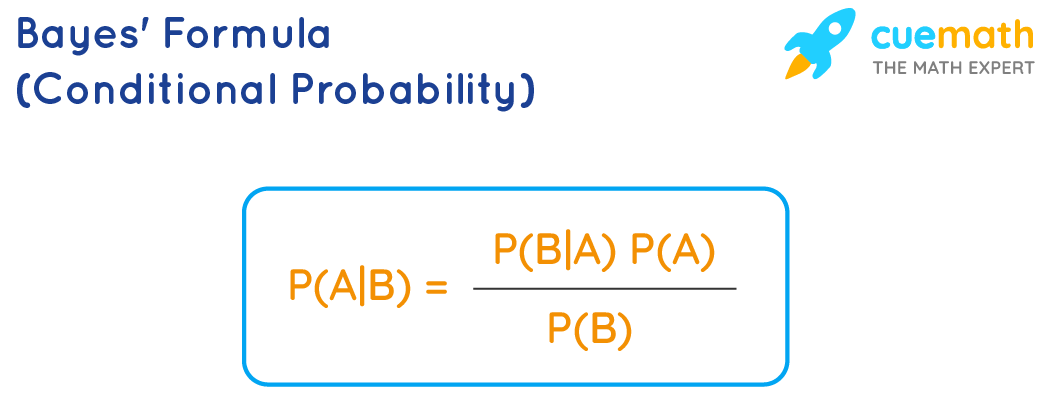# Baye's Formula

Bayes’ formula describes the probability of occurrence of an event in relation to any condition. It is useful for the case of conditional probability. Conditional probability is the likelihood of the occurrence of an event, based on the knowledge of the conditions related to the event. Bayes' formula is also known as the formula for the probability of “causes”. As we scroll down, we will discuss Bayes' theorem statement and Bayes' formula with a few solved examples.

## What Is the Bayes' Formula?

Bayes Formula can be given as:

$P(A|B)= [P(B|A). P(A)]/P(B)$

where  P(A) and P(B) are the respective probabilities of events A and B.

P(A|B) is the probability of event A, given that event B occurs or has occurred.

P(B|A) is the probability of event B, given that event B occurs or has occurred.## Solved Examples Using Bayes' Formula

### Example 1: Jar-1 contains 4 white and 6 black balls while Jar-2 contains 4 white and 3 black balls. One ball is drawn randomly from one of the jars, and was found to be black. Find the probability that it was drawn from the jar-1.

Solution:

Let E1 be the event of choosing jar-1, E2 the event of choosing jar-2, and A be the event of drawing a black ball.

Then, P(E1) = P(E2) = 1/2

Also, P(A|E1) = P(drawing a black ball from Jar-1) = 6/10 = 3/5

P(A|E2) = P(drawing a black ball from Jar-2) = 3/7

By using Bayes’ theorem, we can know the probability of drawing a black ball from the Jar-1 out of two bags,

$$P(E1 \vert A) = \dfrac{P(E_1) \times P(A \vert E_1)}{P(E_1) \times P(A \vert E_1) + P(E_2) \times P(A \vert E_2)}$$

=$$\dfrac{\dfrac{1}{2} \times \dfrac{3}{5}}{\dfrac{1}{2} \times \dfrac{3}{5} + \dfrac{1}{2} \times \dfrac{3}{7}}$$ = 7/12

Answer: The probability of it being drawn from Jar-1 is 7/12.

### Example 2: A man speaks the truth 2 out of 3 times. He reported that the number obtained is a four after throwing a dice. What is the probability that the number obtained is really a four?

Solution:

Let us assume that A is the event of the man reporting that number four is obtained.

Let E1 = event that four is obtained

E2 = complimentary event.

Then, P(E1) = Probability that really four occurs = 1/6

P(E2) = Probability that four not occuring = 1 – P(E1) = 1 −1/6 = 5/6

Also, P(A|E1) = Probability that man reports four and it is really a four = 2/3

P(A|E2) = Probability that man reports four and is false = 1/3

By using Bayes’ theorem, we can know the probability that number obtained is actually a four,

$$P(E1 \vert A) = \dfrac{P(E_1) \times P(A \vert E_1)}{P(E_1) \times P(A \vert E_1) + P(E_2) \times P(A \vert E_2)}$$

= $$\dfrac{\dfrac{1}{6} \times \dfrac{2}{3}}{\dfrac{1}{6} \times \dfrac{2}{3} + \dfrac{5}{6} \times \dfrac{1}{3}}$$ = 2/7

Answer: The probability of a real four on the dice is 2/7.